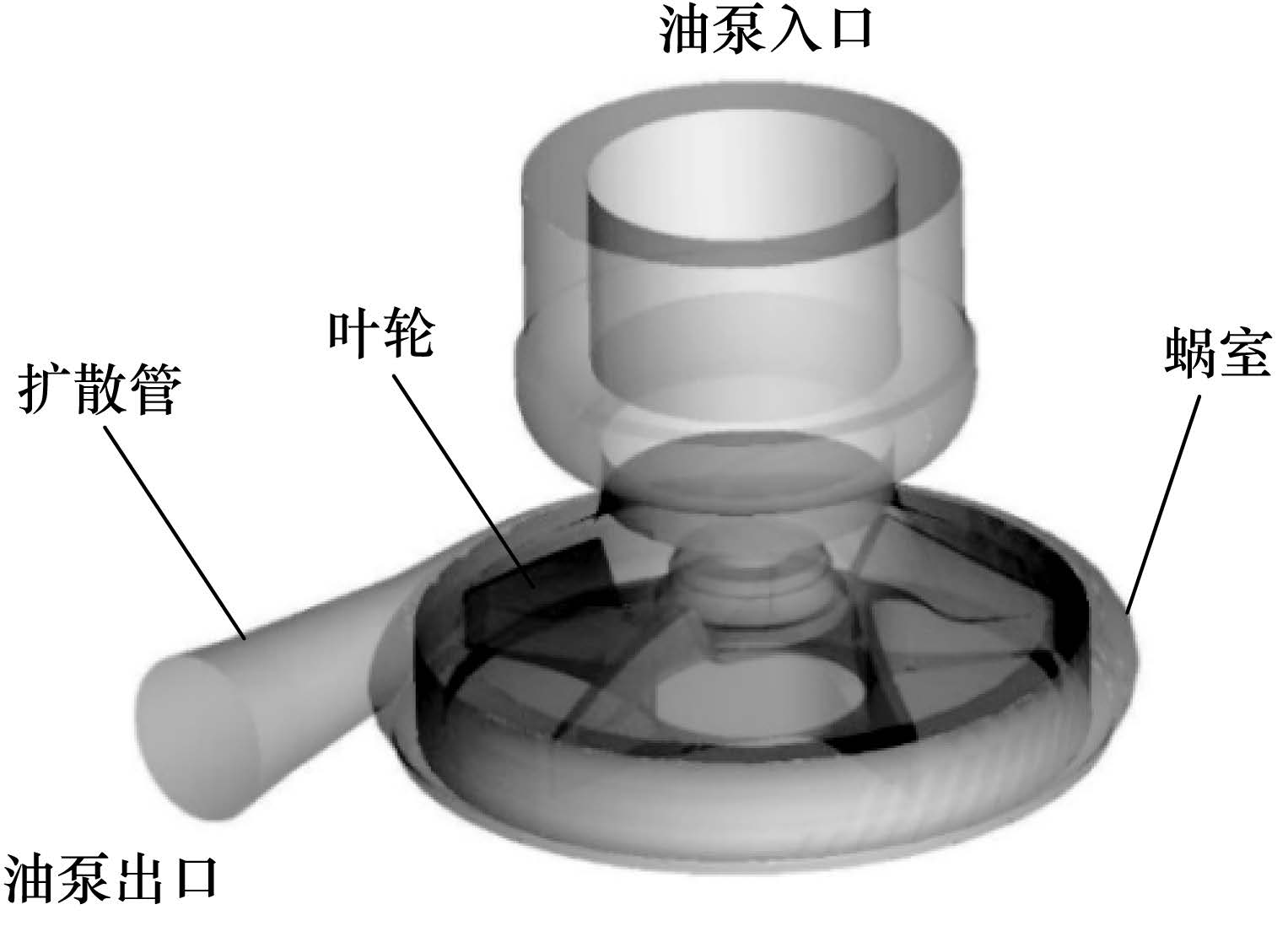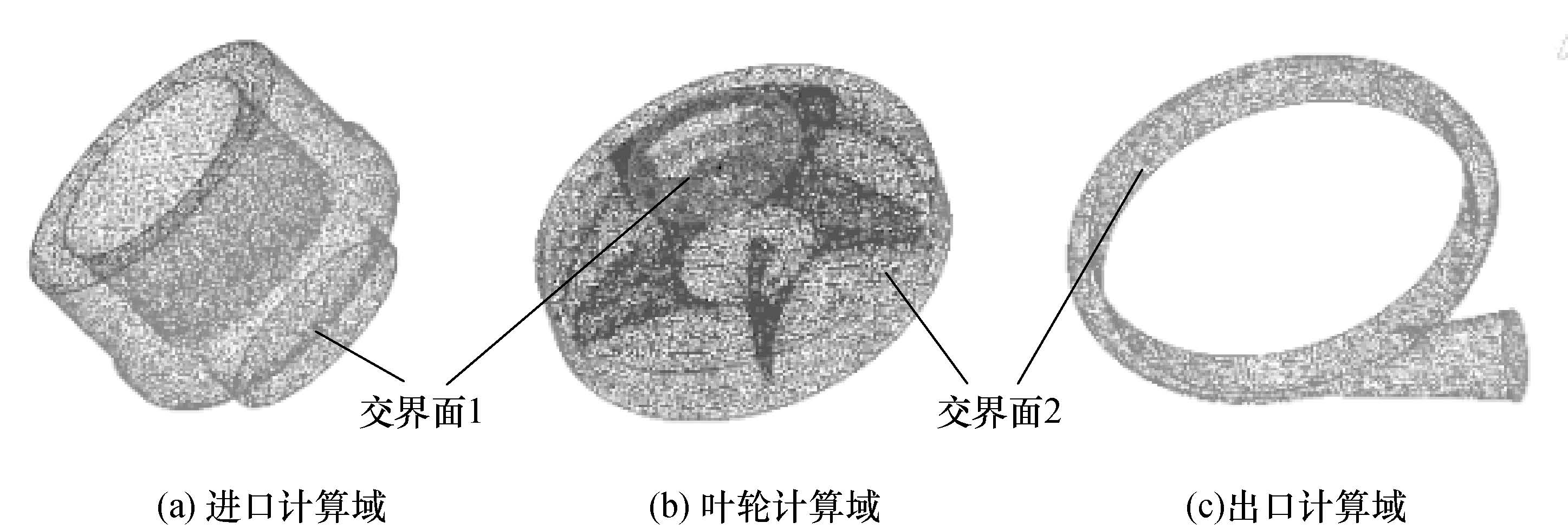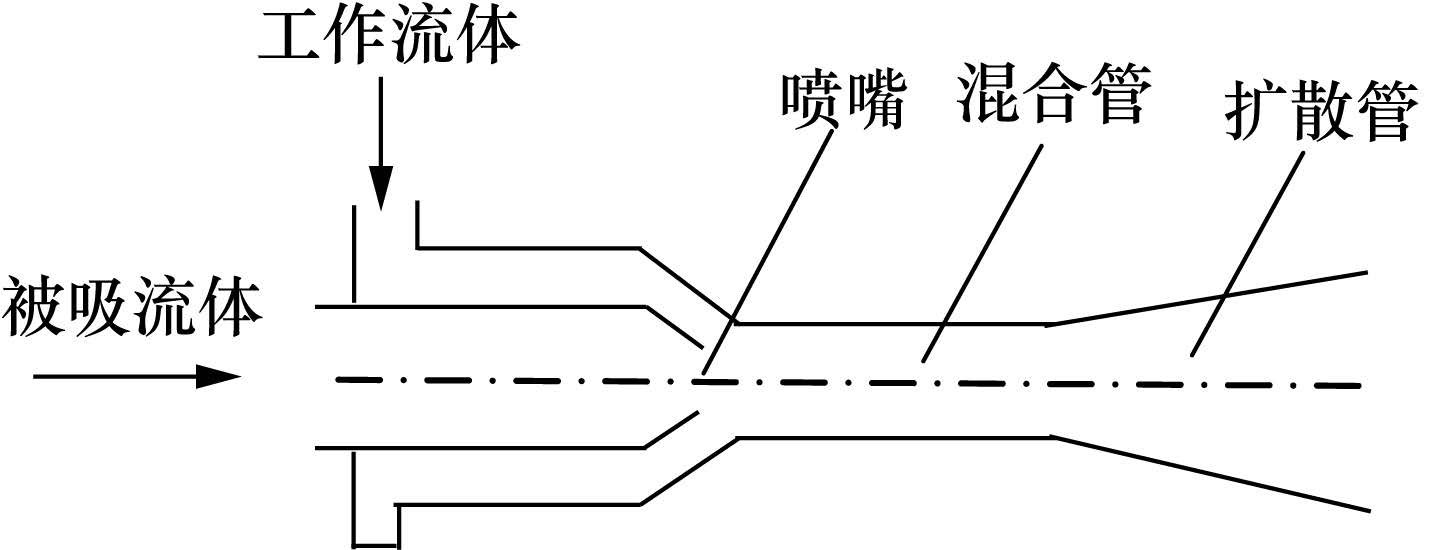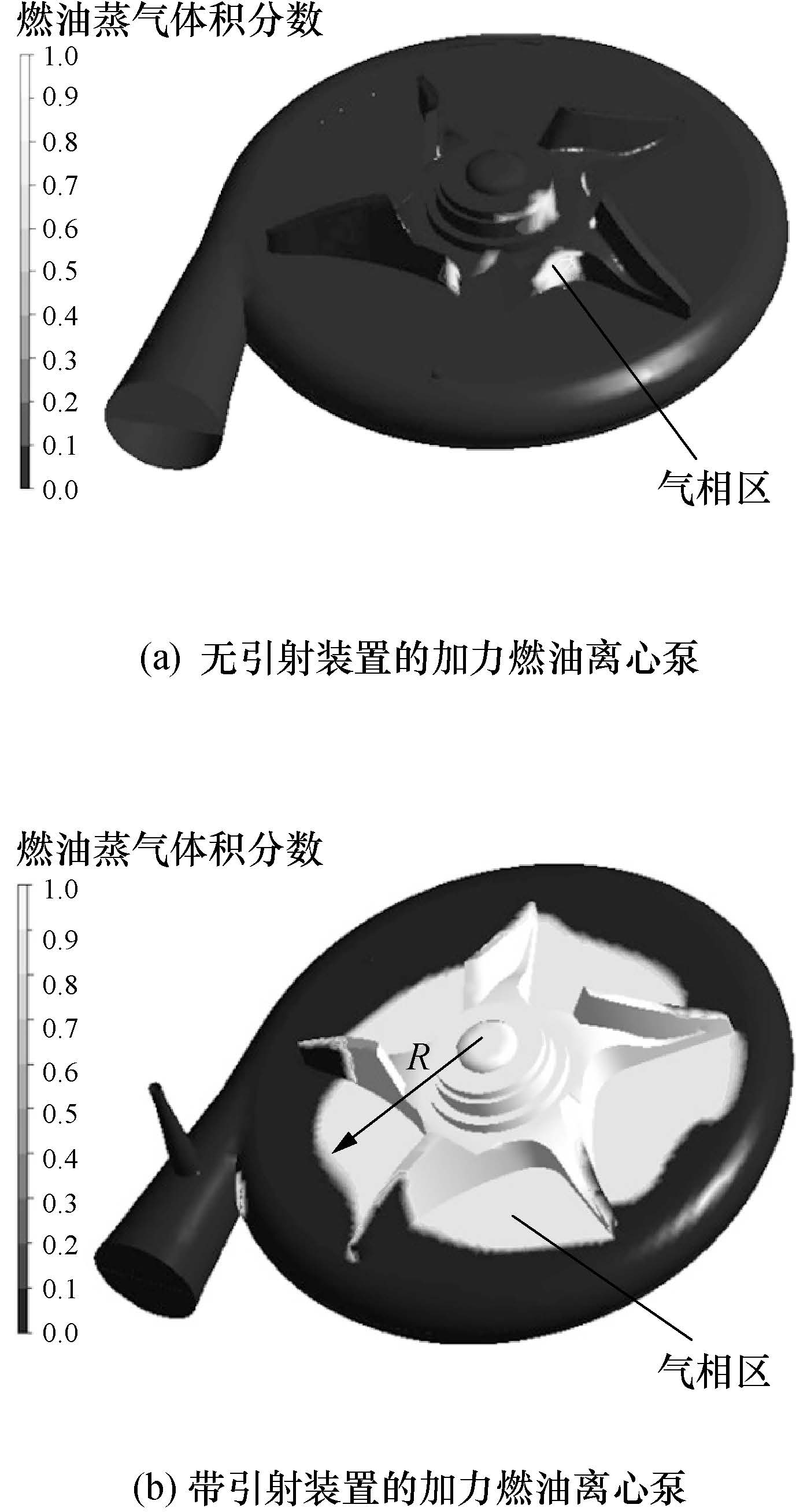1. 清华大学 航天航空学院, 北京 100084 ;
2. 第二炮兵指挥学院, 武汉 430012

Analysis of ejection-cooling at the shut-off operating condition for a reheat fuel centrifugal pump
FAN Hongyu1,2 , LU Qizhi1 , GONG Jingsong1 ,
1. School of Aerospace Engineering, Tsinghua University, Beijing 100084, China ;
2. Second Artillery Command College of PLA, Wuhan 430012, China
Abstract:The high fuel temperature rise at the shut-off operating condition in a reheat fuel centrifugal pump is addressed by cooling the impeller by fuel flowing through a by-pass from the pump inlet. The ejection-cooling is analyzed using the Reynolds average Navier-Strokes (RANS) method with the shear stress transport (SST) turbulence model and the Rayleigh-Plesset cavitation model. The results show that the ejection-cooling design can reduce the high fuel temperature rise at the shut-off condition. The power consumption and the friction heat are both reduced in the reheat fuel centrifugal pump with the ejection-cooling device because of the vapor core region in the impeller central zone which reduces the fuel temperature rise.
Key words: reheat fuel centrifugal pump     shut-off operating condition     ejection-cooling     numerical analysis

1 模型和计算方法 1.1 流体力学模型

 $\frac{\partial \rho }{\partial t}+\text{ }\nabla \cdot \left( \rho V \right)=0,$ (1)
 $\frac{\partial \left( \rho \text{ }V \right)}{\partial t}+\nabla \cdot \left( \rho \text{ }VV \right)=-\nabla p+\mu {{\nabla }^{2}}V+\nabla \cdot \tau .\text{ }$ (2)

1.2 湍流模型

Menter提出的SST湍流模型如下：

 \begin{align} & \frac{D\left( \rho k \right)}{Dt}={{P}_{k}}-{{\beta }^{*}}\rho \text{ }k\omega +\nabla \cdot \left\{ \left( \mu +\text{ }\frac{{{\mu }_{t}}}{{{\sigma }_{k}}} \right)\nabla k \right\}, \\ & \frac{D\left( \rho \omega \right)}{Dt}={{P}_{\omega }}-{{\beta }^{*}}\rho \text{ }k{{\omega }^{2}}+\nabla \cdot \left\{ \left( \mu +\text{ }\frac{{{\mu }_{t}}}{{{\sigma }_{\omega }}} \right)\nabla \omega \right\}+ \\ \end{align} (3)
 $2\left( 1-{{F}_{1}} \right)\text{ }\frac{\rho \text{ }{{\omega }^{2}}}{\omega }\text{ }\nabla k\cdot \text{ }\nabla \omega .$ (4)
 ${{\mu }_{t}}=\min \left[ \rho \text{ }k/\omega ,\rho {{a}_{1}}k/\left( \Omega {{F}_{2}} \right) \right],$ (5)
 $\tau ={{\mu }_{t}}\left( \text{ }\nabla V+\text{ }\nabla {{V}^{T}}\text{ } \right)-\frac{2}{3}kI.$ (6)

1.3 空化模型

 $\frac{({{p}_{B}}-{{p}_{\infty }})}{{{\rho }_{l}}}=R\frac{{{d}^{2}}R}{\text{d}{{t}^{2}}}+\frac{3}{2}{{\left( \frac{\text{d}R}{\text{d}t} \right)}^{2}}+\frac{4\mu }{R}\frac{\text{d}R}{\text{d}t}+\frac{2\sigma }{{{\rho }_{l}}R}.$ (7)

 $\dot{R}[=\frac{\text{d}R}{\text{d}t}=\sqrt{\frac{2}{3}\frac{|{{p}_{B}}-{{p}_{\infty }}|}{{{\rho }_{l}}}}sgn\text{ }\left( {{p}_{B}}-{{p}_{\infty }} \right).\text{ }$ (8)

 $\dot{m}={{\rho }_{g}}4\text{ }\pi \text{ }{{R}^{2}}\dot{R}.$ (9)

 ${{{\dot{m}}}_{lg}}={{\rho }_{g}}4\pi {{R}^{2}}\sqrt{\frac{2}{3}\frac{{{p}_{B}}-{{p}_{\infty }}}{{{\rho }_{l}}}},\text{ }{{p}_{B}}\ge {{p}_{\infty }}.$ (10)

 ${{{\dot{m}}}_{lg}}=-{{\rho }_{g}}4\pi {{R}^{2}}\sqrt{\frac{2}{3}\frac{{{p}_{\infty }}-{{p}_{B}}}{{{\rho }_{l}}}},{{p}_{B}}\le {{p}_{\infty }}.$ (11)

 $\frac{\partial \left( {{\rho }_{g}}{{a}_{g}} \right)}{\partial t}+\nabla \cdot \left( {{\rho }_{g}}{{a}_{g}}V \right)={{{\dot{m}}}_{lg}}+{{{\dot{m}}}_{gl}}.$ (12)

1.4 几何模型图 1 加力燃油离心泵几何模型

1.5 网格与验证图 2 求解计算域网格模型

 网格编号 入口域 叶轮域 出口域 总计 1 41 830 177 472 30 640 249 942 2 236 887 377 147 121 003 735 037 3 236 887 677 763 358 210 1 212 770

 网格编号 α1 α2 α3 1 0.948 9 0.951 2 0.917 6 2 0.952 9 0.961 1 1.035 7 3 0.953 7 0.962 3 1.033 9

2 引射冷却结构设计图 3 两种离心泵结构比较图 4 引射器结构示意图

3 结果与分析图 5 5%额定流量时加力燃油离心泵燃油蒸气体积分数云图

4 结 论

1) 针对加力燃油离心泵关死点工况燃油温升高的问题,设计了引射装置从泵入口引入一小股旁路燃油冷却叶轮腔室,在完成冷却后向低压燃油系统回油。

2) 当加力燃油离心泵的流量为额定流量的5%时,无引射装置的加力燃油离心泵燃油温升为 41.2 ℃,而带引射装置的加力燃油离心泵燃油温升仅为17.5 ℃。

3) 无引射装置的加力燃油离心泵仅在叶轮入口处有少量的气相区,而带引射装置的加力燃油离心泵在叶轮中心出现大范围的气相区。因为在叶轮中心存在气心,使加力燃油离心泵消耗的功率减小,叶轮摩擦产生热量也变小,所以燃油温升降低。

  张天翼. 高速离心泵内流场仿真与减重设计 [D]. 北京: 清华大学, 2011. ZHANG Tianyi. Numerical Simulation of Flow Field and Weight Loss Design for High Speed Centrifugal Pumps [D]. Beijing: Tsinghua University, 2011. (in Chinese)  张绍基. 军用航空发动机燃油与控制系统的研究和发展[J]. 航空发动机,2000, 27 (3) : 14 –27. ZHANG Shaoji. Research and development of fuel and control system for military aeroengine[J]. Aeroengine,2000, 27 (3) : 14 –27. (in Chinese)  刘尚勤. 离心泵用作航空发动机主燃油泵研究[J]. 航空发动机,2006, 33 (2) : 43 –45. LIU Shangqin. Investigation of centrifugal pump used as aeroengine main fuel pump[J]. Aeroengine,2006, 33 (2) : 43 –45. (in Chinese)  关醒凡, 商明华, 张青民. 泵允许的最小运转流量确定方法[J]. 通用机械,2011, 10 (1) : 102 –103. GUAN Xingfan, SHANG Minghua, ZHANG Qingmin. The method for determining pump minimum flow[J]. General Machinery,2011, 10 (1) : 102 –103. (in Chinese)  李培华, 戴云鹤, 曹利, 等. 炉水循环泵电机腔室温升异常的原因分析及对策[J]. 电力科学与工程,2010, 26 (6) : 55 –58. LI Peihua, DAI Yunhe, CAO Li, et al. The analysis for causes and the measures for the abnormal temperature rise in boiler circulating pump motor chamber[J]. Electric Power Science and Engineering,2010, 26 (6) : 55 –58. (in Chinese)  崔虹, 何建设. 泵机组循环试验介质温升分析[J]. 油气储运,2004, 23 (1) : 26 –28. CUI Hong, HE Jianshe. The analysis of medium temperature rise in pump assembly cyclic test[J]. Oil and Gas Storage and Transportation,2004, 23 (1) : 26 –28. (in Chinese)  王福军. 计算流体动力学分析: CFD软件原理及应用[M]. 北京: 清华大学出版社, 2004 . WANG Fujun. Dynamic Analysis of the Computational Fluid Mechanics: CFD Software Principle and Application[M]. Beijing: Tsinghua University Press, 2004 . (in Chinese)  薛梅新. 加力燃油泵隔舌空化机理及抑制方法研究 [D]. 北京: 清华大学, 2012. XUE Meixin. Investigation on Cavitation Mechanism and Suppression Method Close to an Afterburning Fuel Pump Casing Tongue [D]. Beijing: Tsinghua University, 2012. (in Chinese)  Menter F R. Two equation eddy-viscosity turbulence models for engineering applications[J]. AIAA Journal,1994, 32 (8) : 1598 –1605.  Brennen C E. Cavitation and Bubble Dynamics[M]. Oxford: Oxford University Press, 1995 .  Rayleigh O M. On the pressure developed in a liquid during the collapse of a spherical cavity[J]. Philosophical Magazine Series 6,1917, 34 (200) : 94 –98.  Plesset M S. The dynamics of cavitation bubbles[J]. Journal of Applied Mechanics,1949, 16 (3) : 227 –282.  Basker U M. Development of some unconventional centrifugal pumps[J]. Proceedings of the Institution of Mechanical Engineers,1960, 174 (1) : 437 –461.  赵静野, 孙厚钧, 高军. 引射器基本工作原理及其应用[J]. 北京建筑工程学院学报,2001, 16 (4) : 12 –15. ZHAO Jingye, SUN Houjun, GAO Jun. The fundamental theory and employment of ejector mixer[J]. Journal of Beijing Institute of Civil Engineering and Architecture,2001, 16 (4) : 12 –15. (in Chinese)  李立国, 张靖周. 航空用引射混合器[M]. 北京: 国防工业出版社, 2007 . LI Liguo, ZHANG Jingzhou. Aeronautic Ejector Mixer[M]. Beijing: National Defence Industry Press, 2007 . (in Chinese)